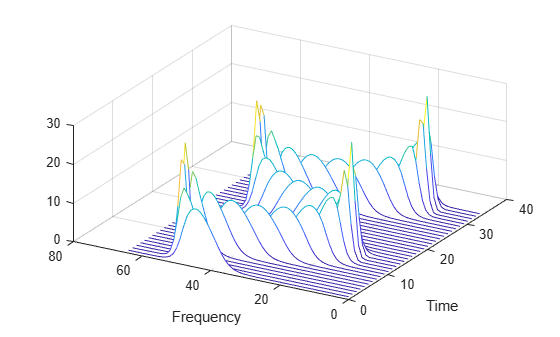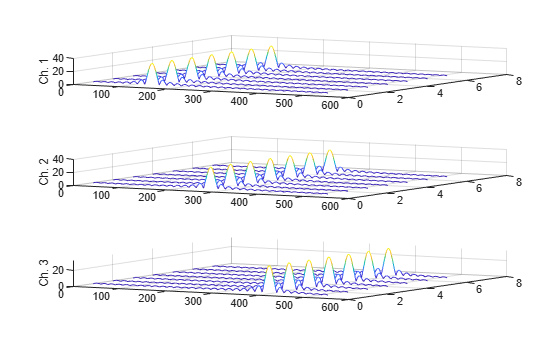stftLayer

Short-time Fourier transform layer

Description

An STFT layer computes the short-time Fourier transform of the input. Use of this layer requires Deep Learning Toolbox™.

Creation

Description

example

layer = stftLayer creates a Short-Time Fourier Transform (STFT) layer. The input to stftLayer must be a dlarray (Deep Learning Toolbox) object in "CBT" format with a size along the time dimension greater than the length of Window.

example

layer = stftLayer(Name=Value) specifies optional parameters using name-value arguments. You can specify the analysis window and the format of the output, among others.

Properties

expand all

STFT

Analysis window used to compute the STFT, specified as a vector with two or more elements.

Example: (1-cos(2*pi*(0:127)'/127))/2 and hann(128) both specify a Hann window of length 128.

Data Types: double | single

Number of overlapped samples, specified as a positive integer strictly smaller than the length of Window.

The stride between consecutive windows is the difference between the window length and the number of overlapped samples.

Data Types: double | single

Number of frequency points used to compute the discrete Fourier transform, specified as a positive integer greater than or equal to the window length. If not specified, this argument defaults to the length of the window.

If the length of the input data along the time dimension is less than the number of DFT points, stftLayer right-pads the data and the window with zeros so they have a length equal to FFTLength.

Data Types: double | single

Layer transform mode, specified as one of these:

• "mag" — STFT magnitude

• "squaremag" — STFT squared magnitude

• "logmag" — Natural logarithm of the STFT magnitude

• "logsquaremag" — Natural logarithm of the STFT squared magnitude

• "realimag" — Real and imaginary parts of the STFT, concatenated along the channel dimension

Data Types: char | string

Layer output mode, specified as one of these:

• "spatiotemporal" — Format the output as a sequence of 1-D images where the image height corresponds to frequency, the second dimension corresponds to channel, the third dimension corresponds to batch, and the fourth dimension corresponds to time.

You can use this output mode to feed the output of stftLayer to a 1-D convolutional layer when you want to convolve along frequency. For more information, see convolution1dLayer (Deep Learning Toolbox).

• "spatial" — Format the output as a sequence of 2-D images where the image height corresponds to frequency and the image width corresponds to time. The third and fourth dimensions correspond to channel and batch, respectively.

You can use this output mode to feed the output of stftLayer to a 2-D convolutional layer when you want to convolve along the two spatial dimensions. For more information, see convolution2dLayer (Deep Learning Toolbox).

• "temporal" — Format the output as a 1-D sequence. This format takes the "spatiotemporal" output format and flattens the image height into the channel dimension. The second dimension of the STFT output corresponds to batch and the third dimension corresponds to time.

You can use this output mode to feed the output of stftLayer to a 1-D convolutional layer when you want to convolve along time. For more information, see convolution1dLayer (Deep Learning Toolbox). You can also use this output mode to use stftLayer as part of a recurrent neural network. For more information, see lstmLayer (Deep Learning Toolbox) and gruLayer (Deep Learning Toolbox).

Data Types: char | string

Layer

Multiplier for weight learning rate, specified as a nonnegative scalar. If not specified, this property defaults to zero, resulting in weights that do not update with training. You can also set this property using the setLearnRateFactor (Deep Learning Toolbox) function.

Data Types: double | single

Layer name, specified as a character vector or a string scalar. For Layer array input, the trainNetwork, assembleNetwork, layerGraph, and dlnetwork functions automatically assign names to layers with Name set to ''.

Data Types: char | string

Number of inputs of the layer. This layer accepts a single input only.

Data Types: double

Input names of the layer. This layer accepts a single input only.

Data Types: cell

Number of outputs of the layer. This layer has a single output only.

Data Types: double

Output names of the layer. This layer has a single output only.

Data Types: cell

Examples

collapse all

Generate a signal sampled at 600 Hz for 2 seconds. The signal consists of a chirp with sinusoidally varying frequency content. Store the signal in a deep learning array with "CTB" format.

fs = 6e2;
x = vco(sin(2*pi*(0:1/fs:2)),[0.1 0.4]*fs,fs);

dlx = dlarray(x,"CTB");

Create a short-time Fourier transform layer with default properties. Create a dlnetwork object consisting of a sequence input layer and the short-time Fourier transform layer. Specify a minimum sequence length of 128 samples. Run the signal through the predict method of the network.

ftl = stftLayer;

dlnet = dlnetwork([sequenceInputLayer(1,MinLength=128) ftl]);
netout = predict(dlnet,dlx);

Convert the network output to a numeric array. Use the squeeze function to remove the length-1 channel and batch dimensions. Plot the magnitude of the STFT. The first dimension of the array corresponds to frequency and the second to time.

q = extractdata(netout);

waterfall(squeeze(q)')
set(gca,XDir="reverse",View=[30 45])
xlabel("Frequency")
ylabel("Time")Generate a 3 × 160 (× 1) array containing one batch of a three-channel, 160-sample sinusoidal signal. The normalized sinusoid frequencies are π/4 rad/sample, π/2 rad/sample, and 3π/4 rad/sample. Save the signal as a dlarray, specifying the dimensions in order. dlarray permutes the array dimensions to the "CBT" shape expected by a deep learning network.

nch = 3;
N = 160;
x = dlarray(cos(pi.*(1:nch)'/4*(0:N-1)),"CTB");

Create a short-time Fourier transform layer that can be used with the sinusoid. Specify a 64-sample rectangular window, 48 samples of overlap between adjoining windows, and 1024 DFT points. Specify the layer output mode as "spatial". By default, the layer outputs the magnitude of the STFT.

stfl = stftLayer(Window=rectwin(64), ...
OverlapLength=48, ...
FFTLength=1024, ...
OutputMode="spatial");

Create a two-layer dlnetwork object containing a sequence input layer and the STFT layer you just created. Treat each channel of the sinusoid as a feature. Specify the signal length as the minimum sequence length for the input layer.

layers = [sequenceInputLayer(nch,MinLength=N) stfl];
dlnet = dlnetwork(layers);

Run the sinusoid through the forward method of the network.

dataout = forward(dlnet,x);

Convert the network output to a numeric array. Use the squeeze function to collapse the size-1 batch dimension. Plot the STFT magnitude separately for each channel in a waterfall plot.

q = squeeze(extractdata(dataout));

for kj = 1:nch
subplot(nch,1,kj)
waterfall(q(:,:,kj)')
view(30,45)
zlabel("Ch. "+string(kj))
endexpand all